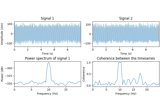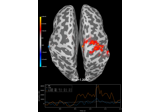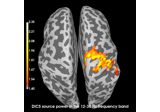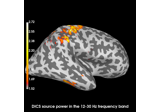# mne.beamformer.make_dics#

mne.beamformer.make_dics(info, forward, csd, reg=0.05, noise_csd=None, label=None, pick_ori=None, rank=None, weight_norm=None, reduce_rank=False, depth=1.0, real_filter=True, inversion='matrix', verbose=None)[source]#

Compute a Dynamic Imaging of Coherent Sources (DICS) spatial filter.

This is a beamformer filter that can be used to estimate the source power at a specific frequency range . It does this by constructing a spatial filter for each source point. The computation of these filters is very similar to those of the LCMV beamformer (`make_lcmv()`), but instead of operating on a covariance matrix, the CSD matrix is used. When applying these filters to a CSD matrix (see `apply_dics_csd()`), the source power can be estimated for each source point.

Parameters:
info`mne.Info`

The `mne.Info` object with information about the sensors and methods of measurement.

forwardinstance of `Forward`

Forward operator.

csdinstance of `CrossSpectralDensity`

The data cross-spectral density (CSD) matrices. A source estimate is performed for each frequency or frequency-bin defined in the CSD object.

reg`float`

The regularization to apply to the cross-spectral density before computing the inverse.

noise_csdinstance of `CrossSpectralDensity` | `None`

Noise cross-spectral density (CSD) matrices. If provided, whitening will be done. The noise CSDs need to have been computed for the same frequencies as the data CSDs. Providing noise CSDs is mandatory if you mix sensor types, e.g. gradiometers with magnetometers or EEG with MEG.

New in v0.20.

label

Restricts the solution to a given label.

pick_ori

For forward solutions with fixed orientation, None (default) must be used and a scalar beamformer is computed. For free-orientation forward solutions, a vector beamformer is computed and:

• `None`

Orientations are pooled after computing a vector beamformer (Default).

• `'normal'`

Filters are computed for the orientation tangential to the cortical surface.

• `'max-power'`

Filters are computed for the orientation that maximizes power.

rank`None` | ‘info’ | ‘full’ | `dict`

This controls the rank computation that can be read from the measurement info or estimated from the data. When a noise covariance is used for whitening, this should reflect the rank of that covariance, otherwise amplification of noise components can occur in whitening (e.g., often during source localization).

`None`

The rank will be estimated from the data after proper scaling of different channel types.

`'info'`

The rank is inferred from `info`. If data have been processed with Maxwell filtering, the Maxwell filtering header is used. Otherwise, the channel counts themselves are used. In both cases, the number of projectors is subtracted from the (effective) number of channels in the data. For example, if Maxwell filtering reduces the rank to 68, with two projectors the returned value will be 66.

`'full'`

The rank is assumed to be full, i.e. equal to the number of good channels. If a `Covariance` is passed, this can make sense if it has been (possibly improperly) regularized without taking into account the true data rank.

`dict`

Calculate the rank only for a subset of channel types, and explicitly specify the rank for the remaining channel types. This can be extremely useful if you already know the rank of (part of) your data, for instance in case you have calculated it earlier.

This parameter must be a dictionary whose keys correspond to channel types in the data (e.g. `'meg'`, `'mag'`, `'grad'`, `'eeg'`), and whose values are integers representing the respective ranks. For example, `{'mag': 90, 'eeg': 45}` will assume a rank of `90` and `45` for magnetometer data and EEG data, respectively.

The ranks for all channel types present in the data, but not specified in the dictionary will be estimated empirically. That is, if you passed a dataset containing magnetometer, gradiometer, and EEG data together with the dictionary from the previous example, only the gradiometer rank would be determined, while the specified magnetometer and EEG ranks would be taken for granted.

The default is `None`.

New in v0.17.

weight_norm

Can be:

• `None`

The unit-gain LCMV beamformer  will be computed.

• `'unit-noise-gain'`

The unit-noise gain minimum variance beamformer will be computed (Borgiotti-Kaplan beamformer) , which is not rotation invariant when `pick_ori='vector'`. This should be combined with `stc.project('pca')` to follow the definition in .

• `'nai'`

The Neural Activity Index  will be computed, which simply scales all values from `'unit-noise-gain'` by a fixed value.

• `'unit-noise-gain-invariant'`

Compute a rotation-invariant normalization using the matrix square root. This differs from `'unit-noise-gain'` only when `pick_ori='vector'`, creating a solution that:

1. Is rotation invariant (`'unit-noise-gain'` is not);

2. Satisfies the first requirement from  that `w @ w.conj().T == I`, whereas `'unit-noise-gain'` has non-zero off-diagonals; but

3. Does not satisfy the second requirement that `w @ G.T = θI`, which arguably does not make sense for a rotation-invariant solution.

Defaults to `None`, in which case no normalization is performed.

reduce_rankbool

If True, the rank of the denominator of the beamformer formula (i.e., during pseudo-inversion) will be reduced by one for each spatial location. Setting `reduce_rank=True` is typically necessary if you use a single sphere model with MEG data.

Changed in version 0.20: Support for reducing rank in all modes (previously only supported `pick='max_power'` with weight normalization).

depth

How to weight (or normalize) the forward using a depth prior. If float (default 0.8), it acts as the depth weighting exponent (`exp`) to use None is equivalent to 0, meaning no depth weighting is performed. It can also be a `dict` containing keyword arguments to pass to `mne.forward.compute_depth_prior()` (see docstring for details and defaults). This is effectively ignored when `method='eLORETA'`.

Changed in version 0.20: Depth bias ignored for `method='eLORETA'`.

real_filterbool

If `True`, take only the real part of the cross-spectral-density matrices to compute real filters.

Changed in version 0.23: Version 0.23 an earlier used `real_filter=False` as the default, as of version 0.24 `True` is the default.

inversion‘single’ | ‘matrix’

This determines how the beamformer deals with source spaces in “free” orientation. Such source spaces define three orthogonal dipoles at each source point. When `inversion='single'`, each dipole is considered as an individual source and the corresponding spatial filter is computed for each dipole separately. When `inversion='matrix'`, all three dipoles at a source vertex are considered as a group and the spatial filters are computed jointly using a matrix inversion. While `inversion='single'` is more stable, `inversion='matrix'` is more precise. See section 5 of . Defaults to `'matrix'`.

Changed in version 0.21: Default changed to `'matrix'`.

verbose

Control verbosity of the logging output. If `None`, use the default verbosity level. See the logging documentation and `mne.verbose()` for details. Should only be passed as a keyword argument.

Returns:
filtersinstance of `Beamformer`

Dictionary containing filter weights from DICS beamformer. Contains the following keys:

‘kind’str

The type of beamformer, in this case ‘DICS’.

‘weights’ndarray, shape (n_frequencies, n_weights)

For each frequency, the filter weights of the beamformer.

‘csd’instance of CrossSpectralDensity

The data cross-spectral density matrices used to compute the beamformer.

‘ch_names’list of str

Channels used to compute the beamformer.

‘proj’ndarray, shape (n_channels, n_channels)

Projections used to compute the beamformer.

‘vertices’list of ndarray

Vertices for which the filter weights were computed.

‘n_sources’int

Number of source location for which the filter weight were computed.

‘subject’str

The subject ID.

‘pick-ori’None | ‘max-power’ | ‘normal’ | ‘vector’

The orientation in which the beamformer filters were computed.

‘inversion’‘single’ | ‘matrix’

Whether the spatial filters were computed for each dipole separately or jointly for all dipoles at each vertex using a matrix inversion.

‘weight_norm’None | ‘unit-noise-gain’

The normalization of the weights.

‘src_type’str

Type of source space.

‘source_nn’ndarray, shape (n_sources, 3)

For each source location, the surface normal.

‘is_free_ori’bool

Whether the filter was computed in a fixed direction (pick_ori=’max-power’, pick_ori=’normal’) or not.

‘whitener’None | ndarray, shape (n_channels, n_channels)

Whitening matrix, provided if whitening was applied to the covariance matrix and leadfield during computation of the beamformer weights.

‘max-power-ori’ndarray, shape (n_sources, 3) | None

When pick_ori=’max-power’, this fields contains the estimated direction of maximum power at each source location.

Notes

The original reference is . See  for a tutorial style paper on the topic.

The DICS beamformer is very similar to the LCMV (`make_lcmv()`) beamformer and many of the parameters are shared. However, `make_dics()` and `make_lcmv()` currently have different defaults for these parameters, which were settled on separately through extensive practical use case testing (but not necessarily exhaustive parameter space searching), and it remains to be seen how functionally interchangeable they could be.

The default setting reproduce the DICS beamformer as described in :

```inversion='single', weight_norm=None, depth=1.
```

To use the `make_lcmv()` defaults, use:

```inversion='matrix', weight_norm='unit-noise-gain-invariant', depth=None
```

For more information about `real_filter`, see the supplemental information from .

References

## Examples using `mne.beamformer.make_dics`#DICS for power mapping

DICS for power mappingCompute source level time-frequency timecourses using a DICS beamformer

Compute source level time-frequency timecourses using a DICS beamformerCompute source power using DICS beamformer

Compute source power using DICS beamformerCompute evoked ERS source power using DICS, LCMV beamformer, and dSPM

Compute evoked ERS source power using DICS, LCMV beamformer, and dSPM